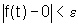Ch 1. Limits Multimedia Engineering Math Limit of aSequence Limit of aFunction LimitLaws Continuity RateChange
 Chapter 1. Limits 2. Derivatives I 3. Derivatives II 4. Mean Value 5. Curve Sketching 6. Integrals 7. Inverse Functions 8. Integration Tech. 9. Integrate App. 10. Parametric Eqs. 11. Polar Coord. 12. Series Appendix Basic Math Units Search eBooks Dynamics Fluids Math Mechanics Statics Thermodynamics Author(s): Hengzhong Wen Chean Chin Ngo Meirong Huang Kurt Gramoll ©Kurt GramollMATHEMATICS - CASE STUDY SOLUTION The thermal conductivity coefficient of a material is a function of temperature. What is the coefficient at the temperature of -∞, 1, +∞. The function is: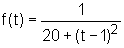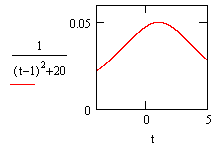Diagram of f(t) when t is from 0 to 5 When t = 1,      f(t) = f(1)           = 1 / [20 + (1 - 1)2]           = 0.05 From the left diagram, it can be found that when t = 1, f(t) is equal to 0.05. The diagram verifies the previous calculated result. Another method to solve this problem is to use the limit of the function.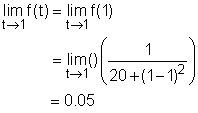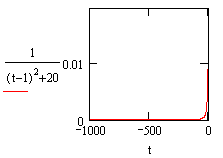Diagram of f(t) when t is from -1000 to 0 When t = -∞,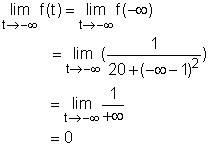Diagram of f(t) when t is from 0 to 1000 When t = +∞,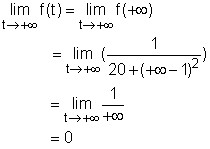Discussion Without limit concept In the case of t approaches -∞ and +∞, when the value of t is substituted into the function equation, the actual quantity of f(t) can not be calculated, because -∞ and +∞ are not actual number. With limit concept As f(t) approaches -∞ ( +∞), for each positive ε, no matter how small, there is a corresponding positive δ, such that if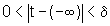then